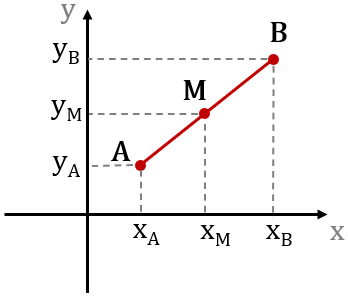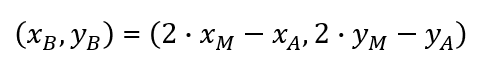# Endpoint Calculator

Use this calculator to determine the coordinates of one endpoint (B) of a line segment determined by its midpoint and its other endpoint (A). Find the endpoint of a line segment.

,
,
Share calculator:

Embed this tool:
get code

## What is an endpoint?

In geometry, an endpoint is one point which defines a segment of a straight line. A segment is defined uniquely by two points (say A and B) and has a unique point (say M) which bisects it (is in the middle). This second property helps when one wants to find one endpoint given the other and the midpoint, as is shown in the formula below.

Each line segment has two endpoints which limit it on each side. In a two-dimensional Cartesian coordinate plane each point has two coordinates - one on each axis, as shown:The point M splits the length of AB in two equal parts. Using an endpoint calculator one can find the coordinates of one endpoint by knowing the coordinates of the other and the midpoint. See our midpoint calculator on how to find the coordinates of the midpoint if the endpoints are known.

## Endpoint formula

The equation for finding the coordinates of one endpoint (B) of a straight line AB given the coordinates of the other (A) and its midpoint M is:where (xA, yA) are the coordinates of point A, (xB, yB) are the coordinates of point B, and (xA, xA) are the coordinates of M. Refer to the illustration above for a more visual presentation. This formula is derived by simply multiplying each side of the midpoint formula by two and then arranging it so that the coordinates we want to find remain on one side of it.

The formula is fairly easy to apply without the help of an endpoint calculator, but using one certainly makes it even faster and easier.

## Endpoints in geometry

An example for using the endpoint formula is if one is given (or has measured) the coordinates of the one endpoint, say (2,6) and of the midpoint, say (3, 12) then by replacing these values in the equations the coordinates of the other endpoint can be determined. The question is, essentially: what is the endpoint B of segment AB, given A and its midpoint?. The calculation is as follows: xM = 2·3 - 2 = 4, yM = 2·12 - 6 = 18. Hence we found the coordinates of the unknown endpoint to be (4, 18).

#### Cite this calculator & page

If you'd like to cite this online calculator resource and information as provided on the page, you can use the following citation:
Georgiev G.Z., "Endpoint Calculator", [online] Available at: https://www.gigacalculator.com/calculators/endpoint-calculator.php URL [Accessed Date: 01 Apr, 2023].